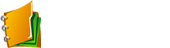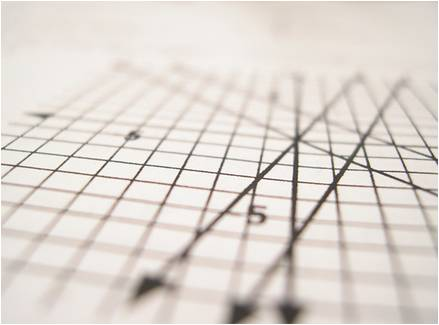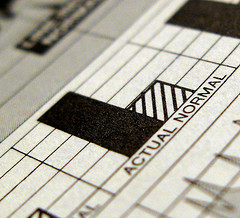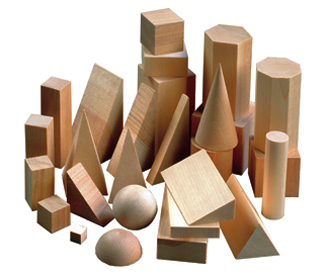﻿ Math Exams and AssignmentsPre-Calculus 12At BCMath.ca we offer hundreds of free online math lessons for students and teachers. Help support our site by clicking on the button above to donate through Paypal.

Assignments

•  Ch 1 Functions and Transformations
•  Ch 2 Sequences and Series
•  Ch 3 Trigonometry and Unit Circles
•  Ch 4 Trigonometric Functions
•  Ch 5 Trigonometric Identities
•  Ch 6 Counting Principles
•  Ch 7 Probability
•  Ch 8 Statistics
•  Ch 9 Relations

Practice Test and Quiz

•  Ch 1 Functions and Transformations
•  Ch 2 Sequences and Series
•  Ch 3 Trigonometry and Unit Circles
•  Ch 4 Trigonometric Functions
•  Ch 5 Trigonometric Identities
•  Ch 6 Counting Principles
•  Ch 7 Probability
•  Ch 8 Statistics
•  Ch 9 RelationsPre-Calculus 11

Assignments

Practice Test and Quiz

•  Ch 1 Sequence and Series
•  Ch 2 Practice Test on Trigonometry
•  Ch 3 Polynomial Functions
•  Ch 4 Rational Functions
•  Ch 5 Systems of Equations
•  Ch 6 Logic and Reasoning
•  Ch 7 Circle Properties
•  Ch 8 Circle Geometry
•  Final Exam Review #1
•  Final Exam Review #2Math 10/11 Honors

Assignments

Practice Test and Quiz

•  Ch 0 Introduction to Functions
•  Ch 2 Sequence and Series
•  Ch 3 Trigonometric Functions & Identities
•  Ch 4 Polynomial Functions
•  Ch 5 Rational Functions and Equations
•  Ch 6 Functions and Transformations
•  Ch 7 Graphing Relations
•  Ch 8 Statistics
•  Final Exam ReviewPre-Calculus & Foundations 10

Solutions to Math Package Review

Practice Test and Quiz

•  Ch 1 Measurement
•  Ch 2 Trigonometry Test
•  Ch 3 Factors and Products
•  Ch 4 Roots and Powers
•  Ch 5 Relations and Functions
•  Ch 6 Linear Functions
•  Ch 7 Systems of Linear EquationsApprenticeship and Workplace 10

Assignments

•  Ch 1 Finance
•  Ch 3 Polynomials
•  Ch 4 Rational Functions
•  Ch 5 Systems of Equations
•  Ch 6 Reasoning and Logic
•  Ch 7 Circle Properties
•  Ch 8 Circle Geometry
•  Ch 9 Coordinate Geometry

Practice Test and Quiz

•  Ch 1 Finance
•  Ch 3 Polynomials
•  Ch 4 Rational Functions
•  Ch 5 Systems of Equations
•  Ch 6 Reasoning and Logic
•  Ch 7 Circle Properties
•  Ch 8 Circle Geometry
•  Ch 9 Coordinate GeometryMath 9/10 Honours

Assignments

Practice Test and Quiz

•  Ch 1 Surface Areas and Square Roots
•  Ch 2 Powers and Exponent Laws
•  Ch 3 Lines and Coorindate Planes
•  Ch 4 Polynomials
•  Ch 5 Inequalities
•  Ch 6 Basic Trigonometric Functions
•  Ch 7 Circle Geometry
•  Ch 8 Introduction to Functions
•  Final Exam ReviewMath 9

Assignments

Practice Test and QuizMath 8/9 Honours

Assignments

Practice Test and Quiz

•  Ch 1 Numeracy and Prime Factorization
•  Ch 2 Percentages and Rates
•  Ch 3 Algebra and Factoring
•  Ch 4 Pythagorean Theorem & Mixed Radicals
•  Ch 5 Triangles and 2D Polygons
•  Ch 6 3D Solids
•  Ch 7 Linear Relations and Graphing Lines
•  Ch 8 Statistics and Probability
•  Ch 9 Tesselations
•  Final Exam Review 1
•  Final Exam Review 2Math 8

Assignments

Practice Test and Quiz